# MCQ in AC Circuits Part 9 | REE Board Exam

(Last Updated On: November 13, 2020)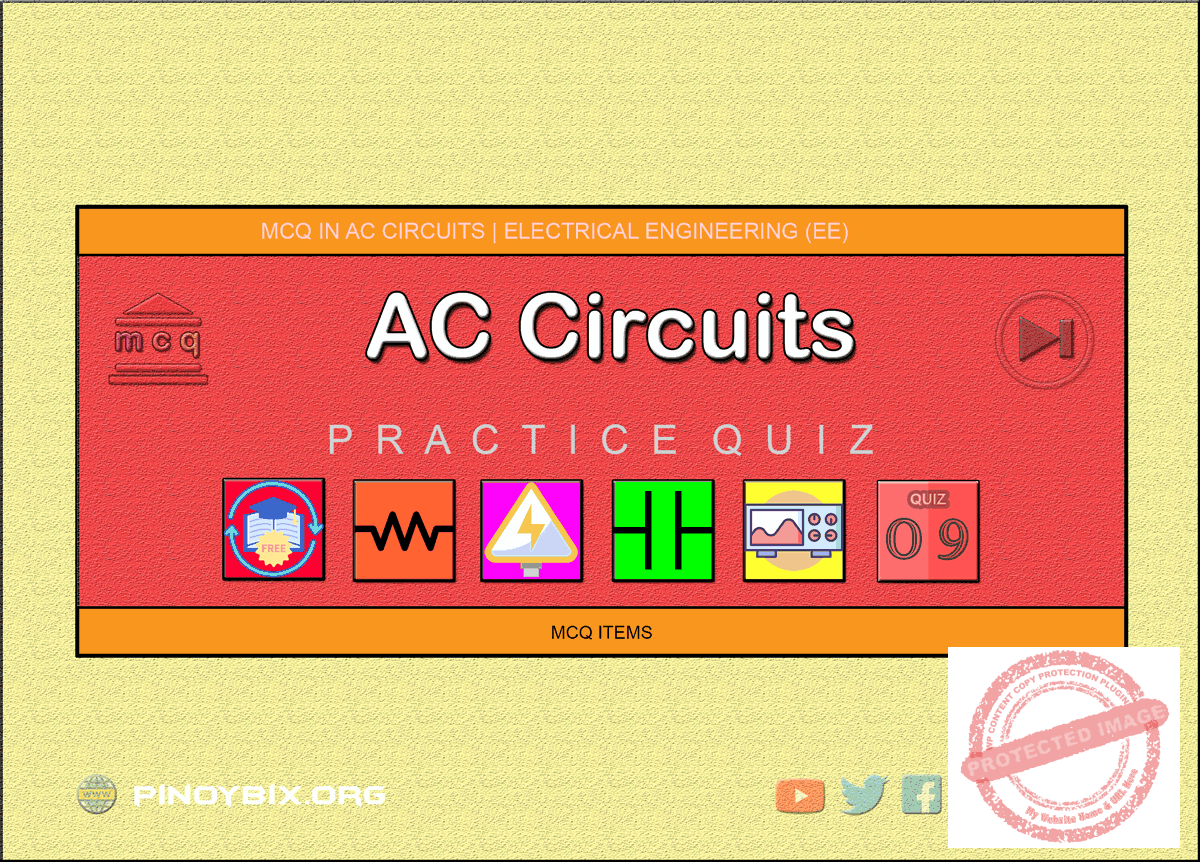This is the Multiple Choice Questions Part 9 of the Series in AC Circuits as one of the Electrical Engineering topic. In Preparation for the REE Board Exam make sure to expose yourself and familiarize in each and every questions compiled here taken from various sources including but not limited to past Board Exam Questions in Electrical Engineering field, Electrical Engineering Books, Journals and other Electrical Engineering References.

#### Continue Practice Exam Test Questions Part 9 of the Series

PARALLEL CIRCUITS

Choose the letter of the best answer in each questions.

401.  The total line current drawn by the circuit shown in Fig. 13.1 is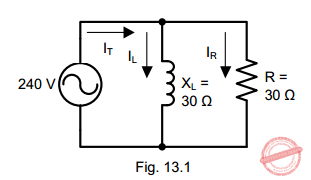A.  8/√2 A

B.  16 A

C.   8√2 A

D.  none of these

Solution:

402.  The power consumed in the circuit shown in Fig. 13.2 is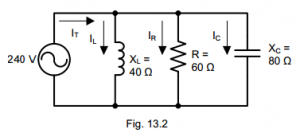A.  480 W

B.  960 W

C.  1200 W

D.  none of these

Solution:

403.  The active component of line current in Fig. 13.2 isA.  6 A

B.  3 A

C.  13 A

D.  4 A

Solution:

404.  The line current drawn by the circuit shown in Fig. 13.2 isA.  13 A

B.  6 A

C.  5 A

D.  none of these

Solution:

405.  The power factor of the circuit shown in Fig. 13.2 isA.  0.8

B.  0.5

C.  0.707

D.  none of these

Solution:

406.  The impedance of the circuit shown in Fig. 13.2 isA.  180 ohms

B.  24 ohms

C.  48 ohms

D.  none of these

Solution:

407.  The circuit shown in Fig. 13.2 isA.  resistive

B.  capacitive

C.  inductive

D.  in resonance

Solution:

408.  If in Fig. 13.2, XL is made equal to XC, the line current will beA.  10 A

B.  6 A

C.  4 A

D.  none of these

Solution:

409.  The power consumed in the circuit shown in Fig. 13.3 is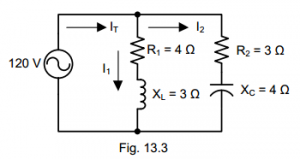A.  8400 W

B.  3600 W

C.  4000 W

D.  none of these

Solution:

410.  If the circuit shown in Fig. 13.3 is connected to 120 V dc, the current drawn by the circuit isA.  24 A

B.  70 A

C.  48 A

D.  30 A

Solution:

411.  The circuit shown in Fig. 13.3 isA.  capacitive

B.  inductive

C.  resistive

D.  in resonance

Solution:

412.  If the source frequency of Fig. 13.4 is low, then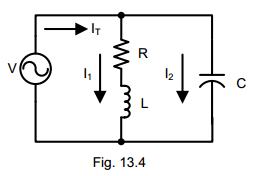A.  coil takes a high lagging current

B.  coil takes a low lagging current

C.  capacitor takes a leading current

D.  circuit offers high impedance

Solution:

413.  If the source frequency of Fig. 13.4 is high, thenA.  coil takes a high lagging current

B.  capacitor takes a high leading current

C.  capacitor takes a low leading current

D.  circuit offers high impedance

Solution:

414.  The circuit shown in Fig. 13.5 is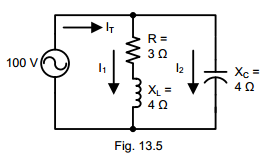A.  in resonance

B.  resistive

C.  inductive

D.  capacitive

Solution:

415.  The circuit shown in Fig. 13.5 will consume a power ofA.  1200 W

B.  2400 W

C.  500 W

D.  none of these

Solution:

416.  If the admittance of a parallel ac circuit is increased, the circuit current

A.  remains constant

B.  is decreased

C.  is increased

D.  none of these

Solution:

417.  The admittance of the circuit shown in Fig. 13.6 is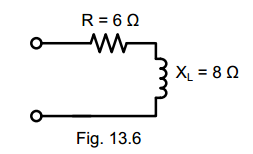A.  10 S

B.  14 S

C.  0.1 S

D.  none of these

Solution:

418.  The conductance of the circuit shown in Fig. 13.6 isA.  14 S

B.  0.6 S

C.  0.06 S

D.  none of these

Solution:

419.  The inductive susceptance of the circuit shown in Fig. 13.6 isA.  8 S

B.  0.8 S

C.  0.08 S

D.  none of these

Solution:

420.  The circuit shown in Fig. 13.7 is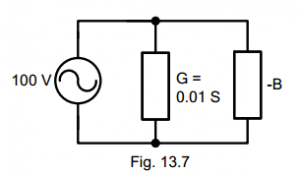A.  resistive

B.  inductive

C.  capacitive

D.  none of these

Solution:

421.  The power loss in the circuit shown in Fig. 13.7 isA.  100 W

B.  10,000 W

C.  10 W

D.  none of these

Solution:

422.  The conductance and susceptance components of admittance are

A.  series elements

B.  parallel elements

C.  series-parallel elements

D.  none of these

Solution:

423.  The  impedance  of  a  circuit  is  10  ohms.  If  the  inductive  susceptance  is  1 siemen, then inductive reactance of the circuit is

A.  10 ohms

B.  1 ohm

C.  100 ohms

D.  none of these

Solution:

424.  The  conductance  and  inductive  susceptance  of  a  circuit  have  the  same magnitude. The power factor of the circuit is

A.  1

B.  0.5

C.  0.707

D.  0.866

Solution:

425.  The admittance of a circuit is (0.1 + j0.8) S. The circuit is

A.  resistive

B.  capacitive

C.  inductive

D.  none of these

Solution:

426.  In a parallel ac circuit, power loss is due to

A.  conductance alone

B.  susceptance alone

C.  both conductance and susceptance

D.  none of these

Solution:

427.  The admittance of a parallel circuit is 0.12∠-30° S. The circuit is

A.  inductive

B.  capacitive

C.  resistive

D.  none of these

Solution:

428.  A circuit have an impedance of (1 –  j2) ohms. The susceptance of the circuit is

A.  0.1 S

B.  0.2 S

C.  0.4 S

D.  none of these

Solution:

429.  A  circuit  has  admittance  of  0.1  S  and  conductance  of  0.08  S.  The  power factor of the circuit is

A.  0.1

B.  0.8

C.  0.08

D.  none of these

Solution:

430.  When an sinusoidal voltage is applied across R-L parallel circuit so that R = XL the phase angle will be

A.  45° lagging

C.  90° lagging

Solution:

431.  In a parallel R-L circuit if IR is the current in resistor and IL is the current in the inductor, then

A.  IR lags IL by 90°

B.  IR leads IL by 270°

C.  IL leads IR by 270°

D.  IL lags IR by 90°

Solution:

432.  The current read by the ammeter A in the ac circuit shown is the given figure is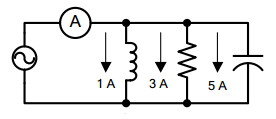A.  9 A

B.  5 A

C.  3 A

D.  1 A

Solution:

433.  In the given figure, the admittance values of the elements in siemens are YR = 0.5 + j0, YL  = 0  –  j1.5 and YC  = 0 + j0.3 respectively. The value of I as a phasor when the voltage E across the elements is 10∠0° V is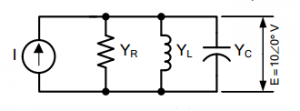A.  1.5 + j-.5

B.  5 – j18

C.  0.5 + j1.8

D.  5 – j12

Solution:

434.  For the circuit shown in the figure, how much the voltage across the inductor leads the voltage across the capacitor?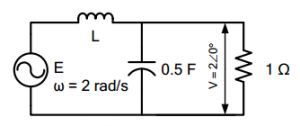A.  45°

B.  90°

C.  135°

D.  180°

Solution:

435.  In the circuit shown in the figure, v = cos 2t, Z2  = 1 + j. C1  is chosen so that i = cos 2t. The value of C1 is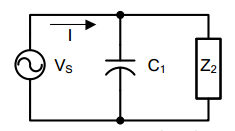A.  2 F

B.  1 F

C.  0.5 F

D.  0.25 F

Solution:

436.  For the given ac circuit, what is the value of I?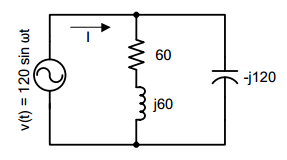A.  1 + j

B.  1 + j0

C.  2 – j

D.  0 + j0

Solution:

437.  For the network shown  in the given figure Z(0) = 3 Ω and Z(∞) = 2 Ω. The values of R1 and R2 will respectively be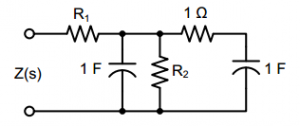A.  2 Ω, 1 Ω

B.  1 Ω, 2 Ω

C.  3 Ω, 2 Ω

D.  2 Ω, 3 Ω

Solution:

438.  The total impedance Z(jω) of the circuit shown is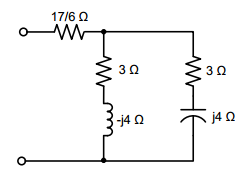A.  6 + j0 Ω

B.  7 + j0 Ω

C.  0 + j8 Ω

D.  6 + j8 Ω

Solution:

439.  A resistance of 40 ohms and an inductive reactor of 30 ohms are joined in parallel to a 120 volts supply as shown in the figure. The power factor of the circuit is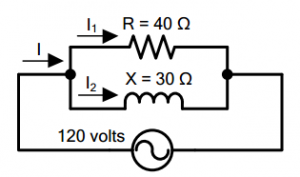A.  0.6

B.  0.7

C.  0.8

D.  unity

Solution:

440.  In a parallel RC circuit,

A.  IC lags IR by 90°

B.  IR and IC are in phase

C.  IC leads IR by 90°

D.  IR leads IC by 90°

Solution:

441.  In a parallel RC circuit,

A.  VC and IR are in phase

B.  VC and IC are in phase

C.  IC and IR are in phase

D.  VC and IR are 90° out of phase

Solution:

442.  When the frequency of the applied voltage increases in a parallel RC circuit

A.  the phase angle, θT, increases

B.  ZEQ increases

C.  ZEQ decreases

D.  both A and C

Solution:

443.  In a parallel RL circuit,

A.  iL lags iR by 90°

B.  iL leads iR by 90°

C.  iL and iR are in phase

D.  iR lags iL by 90°

Solution:

444.  In a parallel RL circuit,

A.  VT and IL are in phase

B.  IL and IR are in phase

C.  VT and IR are in phase

D.  VT and IR are 90° out of phase

Solution:

445.  When the frequency of the applied voltage decreases in a parallel RL circuit

A.  the phase angle, θI, becomes less negative

B.  ZEQ increases

C.  ZEQ decreases

D.  both A and B

Solution:

446.  When the frequency of the applied voltage increases in a parallel RL circuit

A.  θZ increases

B.  ZT decreases

C.  ZT increases

D.  both A and C

Solution:

447.  In an ac circuit with only parallel inductors

A.  IT lags VT by 90°

B.  VT lags IT by 90°

C.  VT and IT are in phase

D.  none of these

Solution:

448.  In a parallel ac circuit with XL and XC

A.  IL and IC are 90° out of phase

B.  IL and IC are in phase

C.  IL and IC are 180° out of phase

D.  XL and XC are 90° out of phase

Solution:

D.  RESONANCE

449.  REE Board Exam October 2000

A series circuit consists of a 20-ohm resistance, a 150 mH inductance and an unknown capacitance. The circuit is supplied with a voltage v = 100 sin 377t. Find the value of capacitance at resonance.

A.  42 μF

B.  47 μF

C.  34.65 μF

D.  72.57 μF

Solution:

450.  REE Board Exam April 2001

A  5  mH  pure  inductance  is  connected  in  parallel  with  one  microfarad capacitor. What frequency will the circuit be antiresonance?

A.  250 Hz

B.  2250 Hz

C.  60 Hz

D.  100 Hz

Solution:

#### Questions and Answers in AC Circuits

Following is the list of practice exam test questions in this brand new series:

MCQ in AC Circuits
PART 1: MCQ from Number 1 – 50                  Answer key: included
PART 2: MCQ from Number 51 – 100              Answer key: included
PART 3: MCQ from Number 101 – 150            Answer key: included
PART 4: MCQ from Number 151 – 200            Answer key: included
PART 5: MCQ from Number 201 – 250             Answer key: included
PART 6: MCQ from Number 251 – 300             Answer key: included
PART 7: MCQ from Number 301 – 350             Answer key: included
PART 8: MCQ from Number 351 – 400             Answer key: included
PART 9: MCQ from Number 401 – 450             Answer key: included
PART 10: MCQ from Number 451 – 500           Answer key: included

P inoyBIX educates thousands of reviewers and students a day in preparation for their board examinations. Also provides professionals with materials for their lectures and practice exams. Help me go forward with the same spirit.

“Will you subscribe today via YOUTUBE?”

Subscribe

PinoyBIX Engineering. © 2014-2020 All Rights Reserved | How to Donate? |